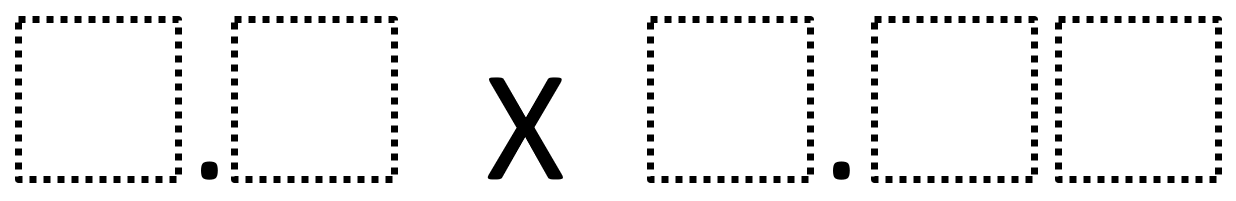Home > Grade 5 > Multiplying Decimals to Make a Whole Number Product

# Multiplying Decimals to Make a Whole Number Product

Directions: Using the digits 1 to 9, at most one time each, fill in the boxes to make a whole number product.### Hint

How can we tell if it is even possible to make a whole number product?
What digits would be better or worse choices for making a whole number product?

The one known answer so far is 9.6 x 8.75 which has a product of 84.

Source: Owen Kaplinsky

## Dividing Whole Numbers With A Decimal Quotient

Directions: Using the digits 1 to 9, at most one time each, place a digit …

1.another one… 9.6 x 1.25 = 12

2.Thanks! I’ll add it to the answer key.

3.Hi
If you think about multiplying integers, you need the product to have a factor of 1000. I.e. you need prime factors of the multipliers to include 5^3 and 2^3. Additionally, the factors of 5 and 2 must stay in separate multipliers otherwise one would have to end in zero which is not allowed.
So possible numbers that would work are:
1.6,2.4,3.2,4.8,5.6,6.4,7.2,9.6
multiplied by
1.25,3.75,6.25,8.75
Any combination of these will work, if the digits are unique.

4.3.75 X 4.8 =18 Also Works

5.Please update answer – I thought there was only one solution then looked in the comments.

6.6.25 x 4.8 = 30

7.1.6 x 3.75 = 6

8.I got an answer of 1.25 x 4.8 = 6

9.6.4 times 1.25 is another answer to get 8… from one of my amazing 5th grade students!